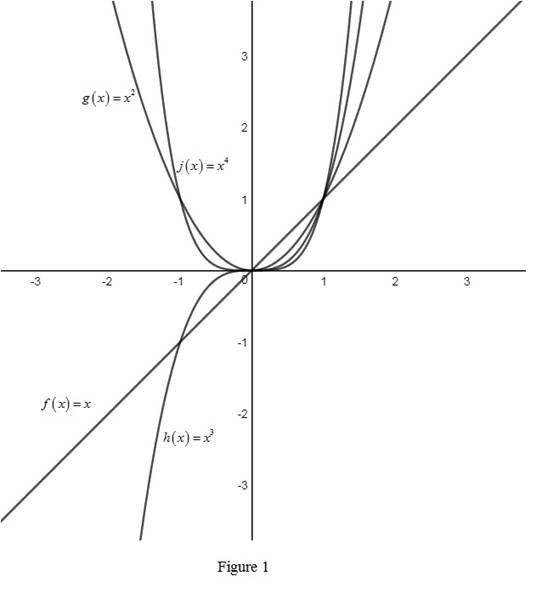# The rough graphs of the function f ( x ) = x , g ( x ) = x 2 , h ( x ) = x 3 and j ( x ) = x 4 on the same axes.### Single Variable Calculus: Concepts...

4th Edition
James Stewart
Publisher: Cengage Learning
ISBN: 9781337687805### Single Variable Calculus: Concepts...

4th Edition
James Stewart
Publisher: Cengage Learning
ISBN: 9781337687805

#### Solutions

Chapter 1, Problem 7RCC
To determine

## To sketch: The rough graphs of the function f(x)=x,g(x)=x2,h(x)=x3 and j(x)=x4 on the same axes.

Expert Solution

### Explanation of Solution

The rough graphs of the functions f(x)=x,g(x)=x2,h(x)=x3 and j(x)=x4 are drawn and shown below in Figure 1.From Figure 1, it is observed that the graphs of the functions g(x)=x2 and j(x)=x4 are symmetric about vertical axis. Therefore, the functions g(x) and j(x) are called even functions as they are symmetric about the origin.

Similarly, it is observed that the graphs of the functions f(x)=xand h(x)=x3 are symmetric about vertical axis. Therefore, the functions f(x) and h(x) are called odd functions as they are symmetric about the origin.

### Have a homework question?

Subscribe to bartleby learn! Ask subject matter experts 30 homework questions each month. Plus, you’ll have access to millions of step-by-step textbook answers!